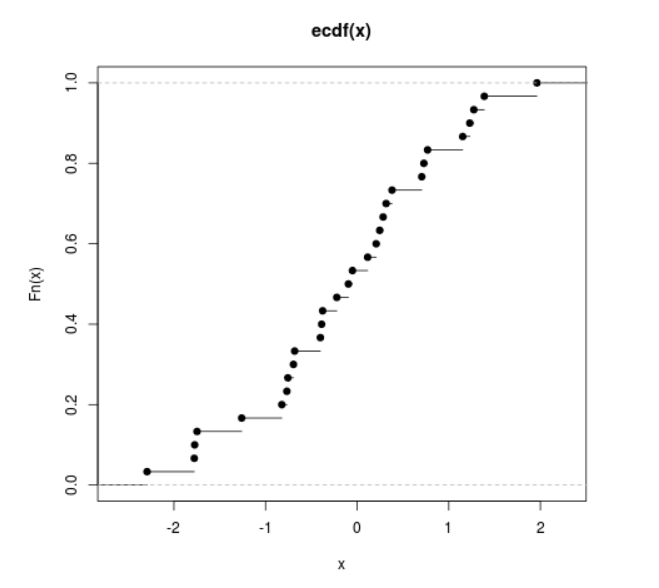Related Articles
Compute the Value of Empirical Cumulative Distribution Function in R Programming – ecdf() Function
• Last Updated : 25 Jun, 2020

`ecdf()` function in R Language is used to compute and plot the value of Empirical Cumulative Distribution Function of a numeric vector.

Syntax: ecdf(x)

Parameters:
x: Numeric Vector

Example 1:

 `# R Program to compute the value of``# Empirical Cumulative Distribution Function`` ` `# Creating a Numeric Vector``x <``-` `seq(``1``, ``50``, by ``=` `2``)`` ` `# Calling ecdf() Function``y <``-` `ecdf(x)``y`

Output:

```Empirical CDF
Call: ecdf(x)
x[1:25] =      1,      3,      5,  ...,     47,     49
```

Example 2:

 `# R Program to compute the value of``# Empirical Cumulative Distribution Function`` ` `# Creating a random vector``x <``-` `rnorm(``30``)`` ` `# Calling ecdf() Function``y <``-` `ecdf(x)`` ` `# Plot a graph``plot(y)`

Output:My Personal Notes arrow_drop_up